# GED Math : Numbers

## Example Questions

← Previous 1 3 4 5 6 7 8 9 18 19

### Example Question #1 : Types Of Numbers And Number Theory

Express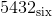as a number in base ten.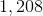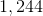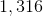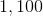Explanation:

Each digit of a base six number has a place value that is a power of six. The lowest four powers of 6, beginning with 1, are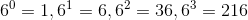, so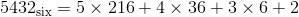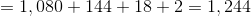### Example Question #1 : Numbers And Operations

How many elements of the set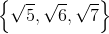are rational?

Three

None

One

Two

None

Explanation:

The square root of an integer is irrational if it is not an integer itself. As can be calculated, all three of these square roots are not integers, so none are rational.

### Example Question #1 : Ged Math

How many elements of the set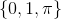are rational?

Two

One

None

Three

Two

Explanation:

0 and 1 are both integers and are therefore rational numbers.is known to be an irrational number, since there are no two integers whose quotient is equal to. "Two" is correct.

### Example Question #1 : Numbers

To how many of the following sets does the number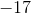belong?

I) The set of whole numbers

II) The set of integers

III) The set of rational numbers

Two

None

One

Three

Two

Explanation:

The whole numbers comprise 0 and the positive integers.is a negative integer, so it belongs to the set of integers, but not the set of whole numbers. Also, all integers are rational by definition, since every integer can be expressed as the quotient of two integers (the integer divided by 1, for example). Therefore,is an integer and a rational number, but not a whole number, and the correct response is two.

### Example Question #1 : Numbers And Operations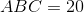., andare integers; they may or may not be distinct.

Which of the following cannot be equal to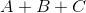?Explanation:

We look for ways to write 20 as the product of three integers, then we find the sum of the integers in each situation. They are: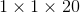Sum: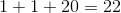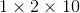Sum: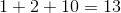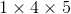Sum: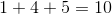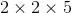Sum: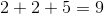9, 10, and 13 are among the possible sums, but 15 is not, so that is the correct response.

### Example Question #1 : Numbers And Operations

To how many of the following sets does the numberbelong?

I) The set of whole numbers

II) The set of integers

III) The set of rational numbers

Three

One

None

Two

Two

Explanation:

The whole numbers comprise 0 and the positive integers.is a negative integer, so it belongs to the set of integers, but not the set of whole numbers. Also, all integers are rational by definition, since every integer can be expressed as the quotient of two integers (the integer divided by 1, for example). Therefore,is an integer and a rational number, but not a whole number, and the correct response is two.

### Example Question #1 : Types Of Numbers And Number Theory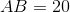.andare integers; they may or may not be distinct.

Which of the following could be equal to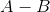?Explanation:

20 can be factored as:

I)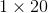II)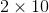III)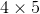The positive difference of the factors can be any of: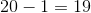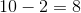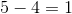Of the four choices, only 8 is possible.

### Example Question #1 : Types Of Numbers And Number Theory

How many subsets does the set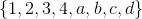have?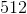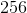Explanation:

The number of subsets of a set withelements is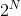, so this eight-element set has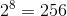subsets.

### Example Question #1 : Types Of Numbers And Number Theory

How many elements are in a set that has exactly 64 subsets?Explanation:

A set withelements hassubsets. Since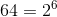, a set with 64 subsets has 6 elements.

### Example Question #1 : Types Of Numbers And Number Theory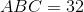, andare distinct integers.

Which of the following could be equal to?Explanation:

We need to find ways to factor 32 such that the three factors are different, and then find the sum of those factors in each case.

32 can be factored as the product of three integers in five differrent ways:

I)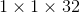II)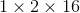III)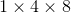IV)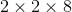V)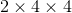Of the five ways, only the second and third involve three distinct factors.

In Case II, the sum of the factors is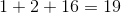.

In Case III, the sum of the factors is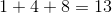.

The only possible correct response is 19.

← Previous 1 3 4 5 6 7 8 9 18 19

### All GED Math Resources